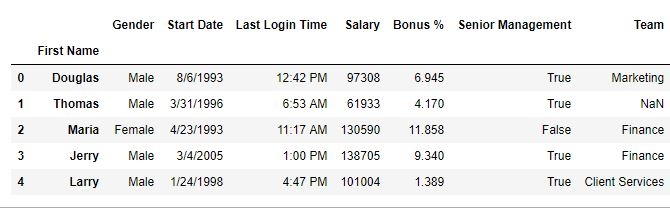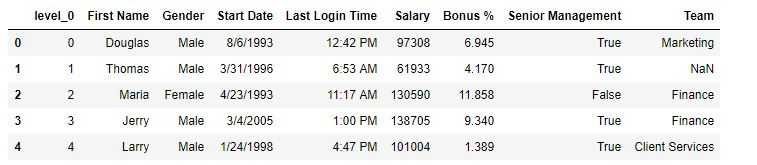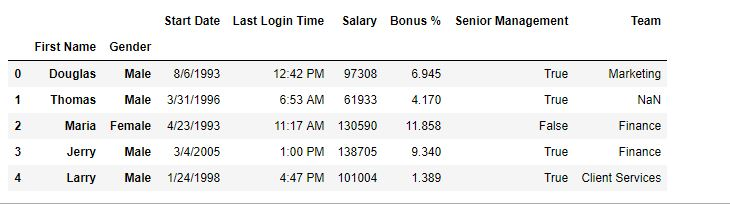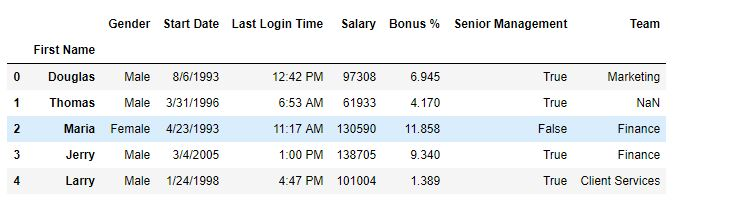Open In App

# Python | Pandas DataFrame.reset_index()

Python is a great language for doing data analysis, primarily because of the fantastic ecosystem of data-centric python packages. Pandas is one of those packages and makes importing and analyzing data much easier.

Pandas `reset_index()` is a method to reset index of a Data Frame. reset_index() method sets a list of integer ranging from 0 to length of data as index.

Syntax:
DataFrame.reset_index(level=None, drop=False, inplace=False, col_level=0, col_fill=”)

Parameters:
level: int, string or a list to select and remove passed column from index.
drop: Boolean value, Adds the replaced index column to the data if False.
inplace: Boolean value, make changes in the original data frame itself if True.
col_level: Select in which column level to insert the labels.
col_fill: Object, to determine how the other levels are named.

Return type: DataFrame

Example #1: Resetting index
In this example, to reset index, First name column have been set as index column first and then using reset index a new index have been generated.

 `# importing pandas package``import` `pandas as pd``  ` `# making data frame from csv file``data ``=` `pd.read_csv(``"employees.csv"``)``  ` `# setting first name as index column``data.set_index([``"First Name"``], inplace ``=` `True``,``                    ``append ``=` `True``, drop ``=` `True``)``  ` `# resetting index``data.reset_index(inplace ``=` `True``)``  ` `# display``data.head()`

Output:
As show in the output images, A new index label named level_0 has been generated.

Before reset –After reset –Example #2: Operation on Multi level Index
In this example, 2 columns(First name and Gender) are added to the index column and later one level is removed by using reset_index() method.

 `# importing pandas package``import` `pandas as pd``  ` `# making data frame from csv file``data ``=` `pd.read_csv(``"employees.csv"``)``  ` `# setting first name as index column``data.set_index([``"First Name"``, ``"Gender"``], inplace ``=` `True``,``                             ``append ``=` `True``, drop ``=` `True``)``  ` `# resetting index``data.reset_index(level ``=` `2``, inplace ``=` `True``, col_level ``=` `1``)``  ` `# display``data.head()`

Output:
As shown in the output image, The gender column in the index column was replaced as it’s level was 2.

Before reset –After reset –